# A simple CNN for the MNIST datasets – II – building the CNN with Keras and a first test

I continue with my series on first exploratory steps with CNNs. After all the discussion of CNN basics in the last article,

A simple CNN for the MNIST datasets – I,

we are well prepared to build a very simple CNN with Keras. By simple I mean simple enough to handle the MNIST digit images. The Keras API for creating CNN models, layers and activation functions is very convenient; a simple CNN does not require much code. So, the Jupyter environment is sufficient for our first experiment.

An interesting topic is the use of a GPU. After a somewhat frustrating experience with a MLP on the GPU of a NV 960 GTX in comparison to a i7 6700K CPU I am eager to see whether we get a reasonable GPU acceleration for a CNN. So, we should prepare our code to use the GPU. This requires a bit of preparation.

We should also ask a subtle question: What do we expect from a CNN in comparison to a MLP regarding the MNIST data? A MLP with 2 hidden layers (with 70 and 30 nodes) provided over 99.5% accuracy on the training data and almost 98% accuracy on a test dataset after some tweaking. Even with basic settings for our MLP we arrived at a value over 97.7% after 35 epochs - below 8 secs. Well, a CNN is probably better in feature recognition than a cluster detection algorithm. But we are talking about the last 2 % of remaining accuracy. I admit that I did not know what to expect ...

# A MLP as an important part of a CNN

At the end of the last article I had discussed a very simple layer structure of convolutional and pooling layers:

• Layer 0: Input layer (tensor of original image data, 3 layers for color channels or one layer for a gray channel)
• Layer 1: Conv layer (small 3x3 kernel, stride 1, 32 filters => 32 maps (26x26), overlapping filter areas)
• Layer 2: Pooling layer (2x2 max pooling => 32 (13x13) maps,
a map node covers 4x4 non overlapping areas per node on the original image)
• Layer 3: Conv layer (3x3 kernel, stride 1, 64 filters => 64 maps (11x11),
a map node covers 8x8 overlapping areas on the original image (total effective stride 2))
• Layer 4: Pooling layer (2x2 max pooling => 64 maps (5x5),
a map node covers 10x10 areas per node on the original image (total effective stride 5), some border info lost)
• Layer 5: Conv layer (3x3 kernel, stride 1, 64 filters => 64 maps (3x3),
a map node covers 18x18 areas per node (effective stride 5), some border info lost )

This is the CNN structure we are going to use in the near future. (Actually, I followed a suggestion of Francois Chollet; see the literature list in the last article). Let us assume that we somehow have established all these convolution and pooling layers for a CNN. Each layer producse some "feature"-related output, structured in form of a tensors. This led to an open question at the end of the last article:

Where and by what do we get a classification of the resulting data with respect to the 10 digit categories of the MNIST images?

Applying filters and extracting "feature hierarchies" of an image alone does not help without a "learned" judgement about these data. But the answer is very simple:

Use a MLP after the last Conv layer and feed it with data from this Conv layer!

When we think in terms of nodes and artificial neurons, we could say: We just have to connect the "nodes" of the feature maps of layer 5 in our special CNN with the nodes of an input layer of a MLP. As a MLP has a flat input layer we need to prepare 9x64 = 576 receiving "nodes" there. We would use weights with a value of "1.0" along these special connections.

Mathematically, this approach can be expressed in terms of a "flattening" operation on the tensor data produced by the the last Conv data. In Numpy terms: We need to reshape the multidimensional tensor containing the values across the stack of maps at the last Conv2D layer into a long 1D array (= a vector).

From a more general perspective we could say: Feeding the output of the Conv part of our CNN into a MLP for classification is quite similar to what we did when we pre-processed the MNIST data by an unsupervised cluster detection algorithm; also there we used the resulting data as input to an MLP. There is one big difference, however:

The optimization of the network's weights during training requires a BW propagation of error terms (more precisely: derivatives of the CNN's loss function) across the MLP AND the convolutional and pooling layers. Error BW propagation should not be stopped at the MLP's input layer: It has to move from the output layer of the MLP back to the MLP's input layer and from there to the convolutional and pooling layers. Remember that suitable filter kernels have to be found during (supervised) training.

If you read my PDF on the error back propagation for a MLP
PDF on the math behind Error Back_Propagation
and think a bit about its basic recipes and results you quickly see that the "input layer" of the MLP is no barrier to error back propagation: The "deltas" discussed in the PDF can be back-propagated right down to the MLP's input layer. Then we just apply the chain rule again. The partial derivatives at the nodes of the input layer with respect to their input values are just "1", because the activation function there is the identity function. The "weights" between the last Conv layer and the input layer of the MLP are no free parameters - we do not need to care about them. And then everything goes its normal way - we apply chain rule after chain rule for all nodes of the maps to determine the gradients of the CNN's loss function with respect to the weights there. But you need not think about the details - Keras and TF2 will take proper care about everything ...

But, you should always keep the following in mind: Whatever we discuss in terms of layers and nodes - in a CNN these are only fictitious interpretations of a series of mathematical operations on tensor data. Not less, not more ..,. Nodes and layers are just very helpful (!) illustrations of non-cyclic graphs of mathematical operations. KI on the level of my present discussion (MLPs, CNNs) "just" corresponds to algorithms which emerge out of a specific deterministic approach to solve an optimization problem.

# Using Tensorflow 2 and Keras

Let us now turn to coding. To be able to use a Nvidia GPU we need a Cuda/Cudnn installation and a working Tensorflow backend for Keras. I have already described the installation of CUDA 10.2 and CUDNN on an Opensuse Linux system in some detail in the article Getting a Keras based MLP to run with Cuda 10.2, Cudnn 7.6 and TensorFlow 2.0 on an Opensuse Leap 15.1 system. You can follow the hints there. In case you run into trouble on your Linux distribution try everything with Cuda 10.1.

Some more hints: TF2 in version 2.2 can be installed by the Pip-mechanism in your virtual Python environment ("pip install --upgrade tensorflow"). TF2 contains already a special Keras version - which is the one we are going to use in our upcoming experiment. So, there is no need to install Keras separately with "pip". Note also that, in contrast to TF1, it is NOT necessary to install a separate package "tensorflow-gpu". If all these things are new to you: You find some articles on creating an adequate ML test and development environment based on Python/PyDev/Jupyter somewhere else in this blog.

# Imports and settings for CPUs/GPUs

We shall use a Jupyter notebook to perform the basic experiments; but I recommend strongly to consolidate your code in Python files of an Eclipse/PyDev environment afterwards. Before you start your virtual Python environment from a Linux shell you should set the following environment variables:

```\$>export OPENBLAS_NUM_THREADS=4 # or whatever is reasonable for your CPU (but do not use all CPU cores and/or hyper threads
\$>export TF_XLA_FLAGS=--tf_xla_cpu_global_jit
\$>export XLA_FLAGS=--xla_gpu_cuda_data_dir=/usr/local/cuda
\$>source bin/activate
(ml_1) \$> jupyter notebook
```

# Required Imports

The following commands in a first Jupyter cell perform the required library imports:

```import numpy as np
import scipy
import time
import sys
import os

import tensorflow as tf
from tensorflow import keras as K
from tensorflow.python.keras import backend as B
from keras import models
from keras import layers
from keras.utils import to_categorical
from keras.datasets import mnist
from tensorflow.python.client import device_lib

from sklearn.preprocessing import StandardScaler
```

Do not ignore the statement "from tensorflow.python.keras import backend as B"; we need it later.

The "StandardScaler" of Scikit-Learn will help us to "standardize" the MNIST input data. This is a step which you should know already from MLPs ... You can, of course, also experiment with different normalization procedures. But in my opinion using the "StandardScaler" is just convenient. ( I assume that you already have installed scikit-learn in your virtual Python environment).

# Settings for CPUs/GPUs

With TF2 the switching between CPU and GPU is a bit of a mess. Not all new parameters and their settings work as expected. As I have explained in the article on the Cuda installation named above, I, therefore, prefer to an old school, but reliable TF1 approach and use the compatibility interface:

```#gpu = False
gpu = True
if gpu:
GPU = True;  CPU = False; num_GPU = 1; num_CPU = 1
else:
GPU = False; CPU = True;  num_CPU = 1; num_GPU = 0

allow_soft_placement=True,
device_count = {'CPU' : num_CPU,
'GPU' : num_GPU},
log_device_placement=True

)
config.gpu_options.per_process_gpu_memory_fraction=0.35
config.gpu_options.force_gpu_compatible = True
B.set_session(tf.compat.v1.Session(config=config))

```

We are brave and try our first runs directly on a GPU. The statement "log_device_placement" will help us to get information about which device - CPU or GP - is actually used.

We prepare a function which loads and prepares the MNIST data for us. The statements reflect more or less what we did with the MNIST dat when we used them for MLPs.

```
# **********
mnist = K.datasets.mnist
(X_train, y_train), (X_test, y_test) = mnist.load_data()

#print(X_train.shape)
#print(X_test.shape)

# preprocess - flatten
len_train =  X_train.shape
len_test  =  X_test.shape
X_train = X_train.reshape(len_train, 28*28)
X_test  = X_test.reshape(len_test, 28*28)

#concatenate
_X = np.concatenate((X_train, X_test), axis=0)
_y = np.concatenate((y_train, y_test), axis=0)

_dim_X = _X.shape

# 32-bit
_X = _X.astype(np.float32)
_y = _y.astype(np.int32)

# normalize
scaler = StandardScaler()
_X = scaler.fit_transform(_X)

# mixing the training indices - MUST happen BEFORE encoding
shuffled_index = np.random.permutation(_dim_X)
_X, _y = _X[shuffled_index], _y[shuffled_index]

# split again
num_test  = 10000
num_train = _dim_X - num_test
X_train, X_test, y_train, y_test = _X[:num_train], _X[num_train:], _y[:num_train], _y[num_train:]

# reshape to Keras tensor requirements
train_imgs = X_train.reshape((num_train, 28, 28, 1))
test_imgs  = X_test.reshape((num_test, 28, 28, 1))
#print(train_imgs.shape)
#print(test_imgs.shape)

# one-hot-encoding
train_labels = to_categorical(y_train)
test_labels  = to_categorical(y_test)
#print(test_labels)

return train_imgs, test_imgs, train_labels, test_labels

if gpu:
with tf.device("/GPU:0"):
else:
with tf.device("/CPU:0"):

```

• Normalization and shuffling: The "StandardScaler" is used for data normalization. I also shuffled the data to avoid any pre-ordered sequences. We know these steps already from the MLP code we built in another article series.
• Image data in tensor form: Something, which is different from working with MLPs is that we have to fulfill some requirements regarding the form of input data. From the last article we know already that our data should have a tensor compatible form; Keras expects data from us which have a certain shape. So, no flattening of the data into a vector here as we were used to with MLPs. For images we, instead, need the width, the height of our images in terms of pixels and also the depth (here 1 for gray-scale images). In addition the data samples are to be indexed along the first tensor axis.
This means that we need to deliver a 4-dimensional array corresponding to a TF tensor of rank 4. Keras/TF2 will do the necessary transformation from a Numpy array to a TF2 tensor automatically for us. The corresponding Numpy shape of the required array is:
(samples, height, width, depth)
Some people also use the term "channels" instead of "depth". In the case of MNIST we reshape the input array - "train_imgs" to (num_train, 28, 28, 1), with "num_train" being the number of samples.
• The use of the function "to_categorical()", more precisely "tf.keras.utils.to_categorical()", corresponds to a one-hot-encoding of the target data. All these concepts are well known from our study of MLPs and MNIST. Keras makes life easy regarding this point ...
• The statements "with tf.device("/GPU:0"):" and "with tf.device("/CPU:0"):" delegate the execution of (suitable) code on the GPU or the CPU. Note that due to the Python/Jupyter environment some code will of course also be executed on the CPU - even if you delegated execution to the GPU.

If you activate the print statements the resulting output should be:

```(60000, 28, 28)
(10000, 28, 28)
(60000, 28, 28, 1)
(10000, 28, 28, 1)
[0. 0. 0. 0. 0. 0. 0. 1. 0. 0.]
```

The last line proves the one-hot-encoding.

# The CNN architecture - and Keras' layer API

Now, we come to a central point: We need to build the 5 central layers of our CNN-architecture. When we build our own MLP code we used a special method to build the different weight arrays, which represented the number of nodes via the array dimensions. A simple method was sufficient as we had no major differences between layers. But with CNNs we have to work with substantially different types of layers. So, how are layers to be handled with Keras?

Well, Keras provides a full layer API with different classes for a variety of layers. You find substantial information on this API and different types of layers at
https://keras.io/api/layers/.

The first section which is interesting for our experiment is https://keras.io/api/ layers/ convolution_layers/ convolution2d/.
You do not need to read much to understand that this is exactly what we need for the "convolutional layers" of our simple CNN model. But how do we instantiate the Conv2D class such that the output works seamlessly together with other layers?

Keras makes our life easy again. All layers are to be used in a purely sequential order. (There are much more complicated layer topologies you can build with Keras! Oh, yes ...). Well, you guess it: Keras offers you a model API; see:
https://keras.io/api/models/.

And there we find a class for a "sequential model" - see https://keras.io/api/ models/sequential/. This class offers us a method "add()" to add layers (and create an instance of the related layer class).

The only missing ingredient is a class for a "pooling" layer. Well, you find it in the layer API documentation, too. The following image depicts the basic structure of our CNN (see the left side of the drawing), as we designed it (see the list above):

# Keras code for the Conv and pooling layers

The convolutional part of the CNN can be set up by the following commands:

Convolutional part of the CNN

```# Sequential layer model of our CNN
# ***********************************

# Build the Conv part of the CNN
# ~~~~~~~~~~~~~~~~~~~~~~~~~~~~~~~

# Choose the activation function for the Conv2D layers
conv_act_func = 1
li_conv_act_funcs = ['sigmoid', 'relu', 'elu', 'tanh']
cact = li_conv_act_funcs[conv_act_func]

# Build the Conv2D layers
cnn = models.Sequential()

```

Easy, isn't it? The nice thing about Keras is that it cares about the required tensor ranks and shapes itself; in a sequential model it evaluates the output of a already defined layer to guess the shape of the tensor data entering the next layer. Thus we have to define an "input_shape" only for data entering the first Conv2D layer!

The first Conv2D layer requires, of course, a shape for the input data. We must also tell the layer interface how many filters and "feature maps" we want to use. In our case we produce 32 maps by first Conv2D layer and 64 by the other two Conv2D layers. The (3x3)-parameter defines the filter area size to be covered by the filter kernel: 3x3 pixels. We define no "stride", so a stride of 1 is automatically used; all 3x3 areas lie close to each other and overlap each other. These parameters result in 32 maps of size 26x26 after the first convolution. The size of the maps of the other layers are given in the layer list at the beginning of this article.

In addition you saw from the code that we chose an activation function via an index of a Python list of reasonable alternatives. You find an explanation of all the different activation functions in the literature. (See also: wikipedia: Activation function). The "sigmoid" function should be well known to you already from my other article series on a MLP.

Now, we have to care about the MLP part of the CNN. The code is simple:

MLP part of the CNN

```# Build the MLP part of the CNN
# ~~~~~~~~~~~~~~~~~~~~~~~~~~~~~~~

# Choose the activation function for the hidden layers of the MLP
mlp_h_act_func = 0
li_mlp_h_act_funcs = ['relu', 'sigmoid', 'tanh']
mhact = li_mlp_h_act_funcs[mlp_h_act_func]

# Choose the output function for the output layer of the MLP
mlp_o_act_func = 0
li_mlp_o_act_funcs = ['softmax', 'sigmoid']
moact = li_mlp_o_act_funcs[mlp_o_act_func]

# Build the MLP layers

```

This all is very straight forward (with the exception of the last statement). The "Flatten"-layer corresponds to the MLP's inout layer. It just transforms the tensor output of the last Conv2D layer into the flat form usable for the first "Dense" layer of the MLP. The first and only "Dense layer" (MLP hidden layer) builds up connections from the flat MLP "input layer" and associates it with weights. Actually, it prepares a weight-tensor for a tensor-operation on the output data of the feeding layer. Dense means that all "nodes" of the previous layer are connected to the present layer's own "nodes" - meaning: setting the right dimensions of the weight tensor (matrix in our case). As a first trial we work with just one hidden layer. (We shall later see that more layers will not improve accuracy.)

I choose the output function (if you will: the activation function of the output layer) as "softmax". This gives us a probability distribution across the classification categories. Note that this is a different approach compared to what we have done so far with MLPs. I shall comment on the differences in a separate article when I find the time for it. At this point I just want to indicate that softmax combined with the "categorical cross entropy loss" is a generalized version of the combination "sigmoid" with "log loss" as we used it for our MLP.

# Parameterization

The above code for creating the CNN would work. However, we want to be able to parameterize our simple CNN. So we include the above statements in a function for which we provide parameters for all layers. A quick solution is to define layer parameters as elements of a Python list - we then get one list per layer. (If you are a friend of clean code design I recommend to choose a more elaborated approach; inject just one parameter object containing all parameters in a structured way. I leave this exercise to you.)

We now combine the statements for layer construction in a function:

```
# Sequential layer model of our CNN
# ***********************************

# just for illustration - th ereal parameters are fed later
# ~~~~~~~~~~~~~~~~~~~~~~~~~~~~~~~~~~~~~~~~~~~~~~~~~~~~~~~~~
li_conv_1   = [32, (3,3), 0]
li_conv_2   = [64, (3,3), 0]
li_conv_3   = [64, (3,3), 0]
li_Conv     = [li_conv_1, li_conv_2, li_conv_3]
li_pool_1   = [(2,2)]
li_pool_2   = [(2,2)]
li_Pool     = [li_pool_1, li_pool_2]
li_dense_1  = [70, 0]
li_dense_2  = [10, 0]
li_MLP      = [li_dense_1, li_dense_2]
input_shape = (28,28,1)

# important !!
# ~~~~~~~~~~~
cnn = None

def build_cnn_simple(li_Conv, li_Pool, li_MLP, input_shape ):

# activation functions to be used in Conv-layers
li_conv_act_funcs = ['relu', 'sigmoid', 'elu', 'tanh']
# activation functions to be used in MLP hidden layers
li_mlp_h_act_funcs = ['relu', 'sigmoid', 'tanh']
# activation functions to be used in MLP output layers
li_mlp_o_act_funcs = ['softmax', 'sigmoid']

# Build the Conv part of the CNN
# ~~~~~~~~~~~~~~~~~~~~~~~~~~~~~~~
num_conv_layers = len(li_Conv)
num_pool_layers = len(li_Pool)
if num_pool_layers != num_conv_layers - 1:
print("\nNumber of pool layers does not fit to number of Conv-layers")
sys.exit()
rg_il = range(num_conv_layers)

# Define a sequential model
cnn = models.Sequential()

for il in rg_il:
num_filters = li_Conv[il]
t_fkern_size = li_Conv[il]
cact        = li_conv_act_funcs[li_Conv[il]]
if il==0:
else:

if il < num_pool_layers:
t_pkern_size = li_Pool[il]

# Build the MLP part of the CNN
# ~~~~~~~~~~~~~~~~~~~~~~~~~~~~~~~
num_mlp_layers = len(li_MLP)
rg_im = range(num_mlp_layers)

for im in rg_im:
n_nodes = li_MLP[im]
if im < num_mlp_layers - 1:
m_act   =  li_mlp_h_act_funcs[li_MLP[im]]
else:
m_act   =  li_mlp_o_act_funcs[li_MLP[im]]

return cnn

```

We return the model "cnn" to be able to use it afterwards.

# How many parameters does our CNN have?

The layers contribute with the following numbers of weight parameters:

• Layer 1: (32 x (3x3)) + 32 = 320
• Layer 3: 32 x 64 x (3x3) + 64 = 18496
• Layer 5: 64 x 64 x (3x3) + 64 = 36928
• MLP : (576 + 1) x 70 + (70 + 1) x 10 = 41100

Making a total of 96844 weight parameters. Our standard MLP discussed in another article series had (784+1) x 70 + (70 + 1) x 30 + (30 +1 ) x 10 = 57390 weights. So, our CNN is bigger and the CPU time to follow and calculate all the partial derivatives will be significantly higher. So, we should definitely expect some better classification data, shouldn't we?

# Compilation

Now comes a thing which is necessary for models: We have not yet defined the loss function and the optimizer or a learning rate. For the latter Keras can choose a proper value itself - as soon as it knows the loss function. But we should give it a reasonable loss function and a suitable optimizer for gradient descent. This is the main purpose of the "compile()"-function.

```cnn.compile(optimizer='rmsprop', loss='categorical_crossentropy', metrics=['accuracy'])
```

Although TF2 can already analyze the graph of tensor operations for partial derivatives, it cannot guess the beginning of the chain rule sequence.

As we have multiple categories "categorial_crossentropy" is a good choice for the loss function. We should also define which optimized gradient descent method is used; we choose "rmsprop" - as this method works well in most cases. A nice introduction is given here: towardsdatascience: understanding-rmsprop-faster-neural-network-learning-62e116fcf29a. But see the books mentioned in the last article on "rmsprop", too.

Regarding the use of different metrics for different tasks see machinelearningmastery.com / custom-metrics-deep-learning-keras-python/. In case of a classification problem, we are interested in the categorical "accuracy". A metric can be monitored during training and will be recorded (besides aother data). We can use it for plotting information on the training process (a topic of the next article).

# Training

Training is done by a function model.fit() - here: cnn.fit(). This function accepts a variety of parameters explained here: https://keras.io/ api/ models/ model_training_apis/ #fit-method.

We now can combine compilation and training in one function:

```# Training
def train( cnn, build=False, train_imgs, train_labels, reset, epochs, batch_size, optimizer, loss, metrics,
li_Conv, li_Poo, li_MLP, input_shape ):
if build:
cnn = build_cnn_simple( li_Conv, li_Pool, li_MLP, input_shape)
cnn.compile(optimizer=optimizer, loss=loss, metrics=metrics)
cnn.save_weights('cnn_weights.h5') # save the initial weights
# reset weights
if reset and not build:
start_t = time.perf_counter()
cnn.fit(train_imgs, train_labels, epochs=epochs, batch_size=batch_size, verbose=1, shuffle=True)
end_t = time.perf_counter()
fit_t = end_t - start_t
return cnn, fit_t  # we return cnn to be able to use it by other functions
```

Note that we save the initial weights to be able to load them again for a new training - otherwise Keras saves the weights as other model data after training and continues with the last weights found. The latter can be reasonable if you want to continue training in defined steps. However, in our forthcoming tests we repeat the training from scratch.

Keras offers a "save"-model and methods to transfer data of a CNN model to files (in two specific formats). For saving weights the given lines are sufficient. Hint: When I specify no path to the file "cnn_weights.h5" the data are - at least in my virtual Python environment - saved in the directory where the notebook is located.

# First test

In a further Jupyter cell we place the following code for a test run:

```
# Perform a training run
# ********************
build = False
if cnn == None:
build = True
batch_size=64
epochs=5
reset = True # we want training to start again with the initial weights

optimizer='rmsprop'
loss='categorical_crossentropy'
metrics=['accuracy']

li_conv_1   = [32, (3,3), 0]
li_conv_2   = [64, (3,3), 0]
li_conv_3   = [64, (3,3), 0]
li_Conv     = [li_conv_1, li_conv_2, li_conv_3]
li_pool_1   = [(2,2)]
li_pool_2   = [(2,2)]
li_Pool     = [li_pool_1, li_pool_2]
li_dense_1  = [70, 0]
li_dense_2  = [10, 0]
li_MLP      = [li_dense_1, li_dense_2]
input_shape = (28,28,1)

try:
if gpu:
with tf.device("/GPU:0"):
cnn, fit_time = train( cnn, build, train_imgs, train_labels,
reset, epochs, batch_size, optimizer, loss, metrics,
li_Conv, li_Pool, li_MLP, input_shape)
print('Time_GPU: ', fit_time)
else:
with tf.device("/CPU:0"):
cnn, fit_time = train( cnn, build, train_imgs, train_labels,
reset, epochs, batch_size, optimizer, loss, metrics,
li_Conv, li_Pool, li_MLP, input_shape)
print('Time_CPU: ', fit_time)
except SystemExit:
print("stopped due to exception")
```

You recognize the parameterization of our train()-function. What results do we get ?

```Epoch 1/5
60000/60000 [==============================] - 4s 69us/step - loss: 0.1551 - accuracy: 0.9520
Epoch 2/5
60000/60000 [==============================] - 4s 69us/step - loss: 0.0438 - accuracy: 0.9868
Epoch 3/5
60000/60000 [==============================] - 4s 68us/step - loss: 0.0305 - accuracy: 0.9907
Epoch 4/5
60000/60000 [==============================] - 4s 69us/step - loss: 0.0227 - accuracy: 0.9931
Epoch 5/5
60000/60000 [==============================] - 4s 69us/step - loss: 0.0184 - accuracy: 0.9948
Time_GPU:  20.610678611003095
```

And a successive check on the test data gives us: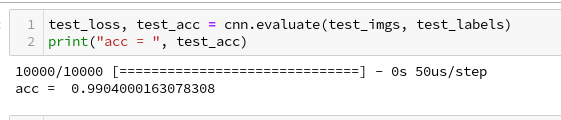We can ask Keras also for a description of the model:

# Accuracy at the 99% level

We got an accuracy on the test data of 99%! With 5 epochs in 20 seconds - on my old GPU.
This leaves us a very good impression - on first sight ...

# Conclusion

We saw today that it is easy to set up a CNN. We used a simple MLP to solve the problem of classification; the data to its input layer are provided by the output of the last convolutional layer. The tensor there has just to be "flattened".

The level of accuracy reached is impressing. Well, its also a bit frustrating when we think about the efforts we put into our MLP, but we also get a sense for the power and capabilities of CNNs.

In the next article
A simple CNN for the MNIST dataset – III – inclusion of a learning-rate scheduler, momentum and a L2-regularizer
we will care a bit about plotting. We at least want to see the same accuracy and loss data which we used to plot at the end of our MLP tests.

# A simple Python program for an ANN to cover the MNIST dataset – XIII – the impact of regularization

I continue with my growing series on a Multilayer perceptron and the MNIST dataset.

In the last article of the series we made some interesting experiences with the variation of the "leaning rate". We also saw that a reasonable range for initial weight values should be chosen.

Even more fascinating was, however, the impact of a normalization of the input data on a smooth and fast gradient descent. We drew the conclusion that normalization is of major importance when we use the sigmoid function as the MLP's activation function - especially for nodes in the first hidden layer and for input data which are on average relatively big. The reason for our concern were saturation effects of the sigmoid functions and other functions with a similar variation with their argument. In the meantime I have tried to make the importance of normalization even more plausible with the help of a a very minimalistic perceptron for which we can analyze saturation effects a bit more in depth; you get to the related article series via the following link:

There we also have a look at other normalizers or feature scalers.

But back to our series on a multi-layer perceptron. You may have have asked yourself in the meantime: Why did he not check the impact of the regularization? Indeed: We kept the parameter Lambda2 for the quadratic regularization term constant in all experiments so far: Lambda2 = 0.2. So, the question about the impact of regularization e.g. on accuracy is a good one.

# How big is the regularization term and how does it evolve during gradient decent training?

I add even one more question: How big is the relative contribution of the regularization term to the total loss or cost function? In our Python program for a MLP model we included a so called quadratic Ridge term:

Lambda2 * 0.5 * SUM[all weights**2], where bias nodes are excluded from the sum.

From various books on Machine Learning [ML] you just learn to choose the factor Lambda2 in the range between 0.01 and 0.1. But how big is the resulting term actually in comparison to the standard cost term, then, and how does the ratio between both terms evolve during gradient descent? What factors influence this ratio?

As we follow a training strategy based on mini-batches the regularization contribution was and is added up to the costs of each mini-batch. So its relative importance varies of course with the size of the mini-batches! Other factors which may also be of some importance - at least during the first epochs - could be the total number of weights in our network and the range of initial weight values.

Regarding the evolution during a converging gradient descent we know already that the total costs go down on the path to a cost minimum - whilst the weight values reach a stable level. So there is a (non-linear!) competition between the regularization term and the real costs of the "Log Loss" cost function! During convergence the relative importance of the regularization term may therefore become bigger until the ratio to the standard costs reaches an eventual constant level. But how dominant will the regularization term get in the end?

Let us do some experiments with the MNIST dataset again! We fix some common parameters and conditions for our test runs:
As we saw in the last article we should normalize the input data. So, all of our numerical experiments below (with the exception of the last one) are done with standardized input data (using Scikit-Learn's StandardScaler). In addition initial weights are all set according to the sqrt(nodes)-rule for all layers in the interval [-0.5*sqrt(1/num_nodes), 0.5*sqrt(1/num_nodes)], with num_nodes meaning the number of nodes in a layer. Other parameters, which we keep constant, are:

Parameters: learn_rate = 0.001, decrease_rate = 0.00001, mom_rate = 0.00005, n_size_mini_batch = 500, n_epochs = 800.

I added some statements to the method for cost calculation in order to save the relative part of the regularization terms with respect to the total costs of each mini-batch in a Numpy array and plot the evolution in the end. The changes are so simple that I omit showing the modified code.

# A first look at the evolution of the relative contribution of regularization to the total loss of a mini-batch

How does the outcome of gradient descent look for standardized input data and a Lambda2-value of 0.1?

Lambda2 = 0.1
Results: acc_train: 0.999 , acc_test: 0.9714, convergence after ca. 600 epochs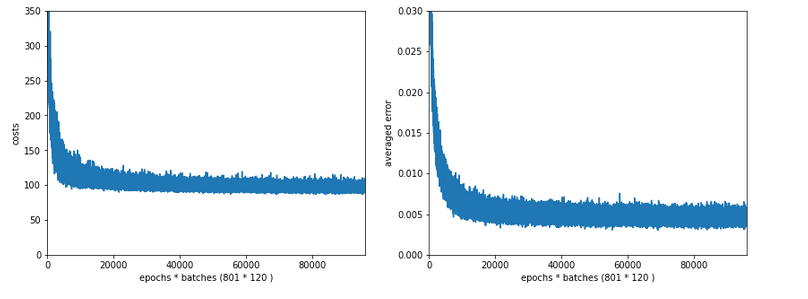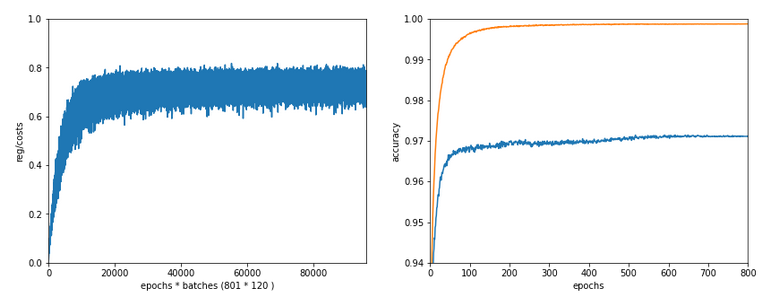We see that the regularization term actually dominates the total loss of a mini-batch at convergence. At least with our present parameter setting. In comparisoin to the total loss of the full training set the contribution is of course much smaller and typically below 1%.

# A small Lambda term

Let us reduce the regularization term via setting Lambda = 0.01. We expect its initial contribution to the costs of a batch to be smaller then, but this does NOT mean that the ratio to the standard costs of the batch automatically shrinks significantly, too:

Lambda2 = 0.01
Results: acc_train: 1.0 , acc_test: 0.9656, convergence after ca. 350 epochs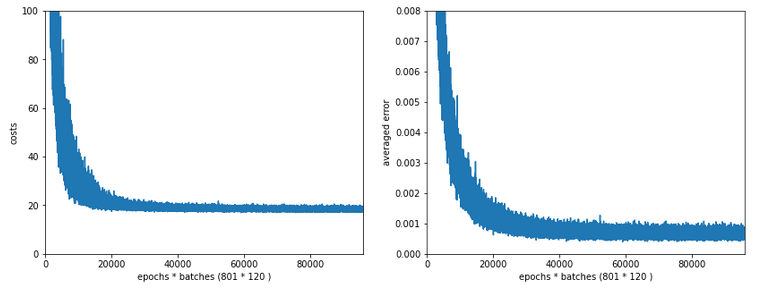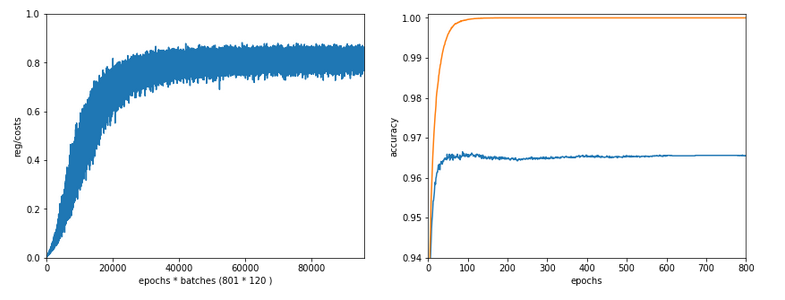Note the absolute scale of the costs in the plots! We ended up at a much lower level of the total loss of a batch! But the relative dominance of regularization at the point of convergence actually increased! However, this did not help us with the accuracy of our MLP-algorithm on the test data set - although we perfectly fit the training set by a 100% accuracy.

In the end this is what regularization is all about. We do not want a total overfitting, a perfect adaption of the grid to the training set. It will not help in the sense of getting a better general accuracy on other input data. A Lambda2 of 0.01 is much too small in our case!

# Slightly bigger regularization with Lambda2 = 0.2

So lets enlarge Lambda2 a bit:
Lambda2 = 0.2
Results: acc_train: 0.9946 , acc_test: 0.9728, convergence after ca. 700 epochs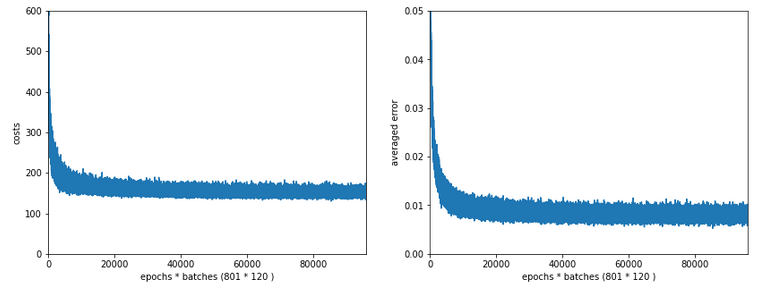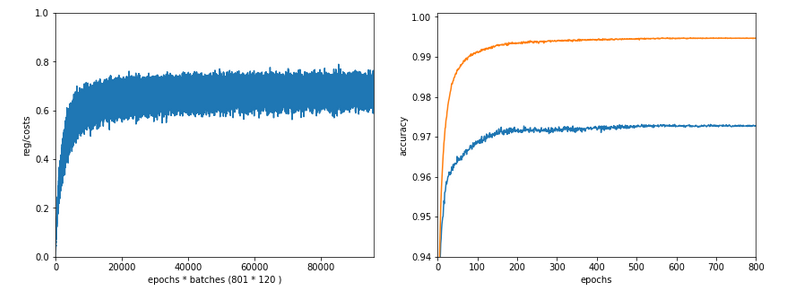We get an improved accuracy!

# Two other cases with significantly bigger Lambda2

Lambda2 = 0.4
Results: acc_train: 0.9858 , acc_test: 0.9693, convergence after ca. 600 epochs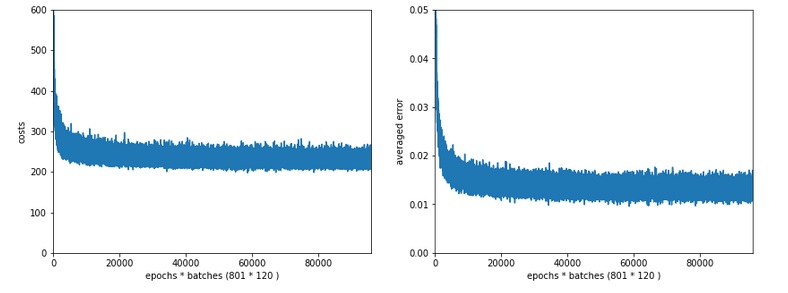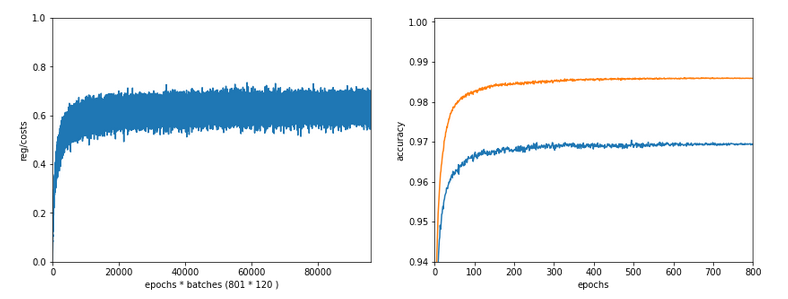Lambda2 = 0.8
Results: acc_train: 0.9705 , acc_test: 0.9588, convergence after ca. 400 epochs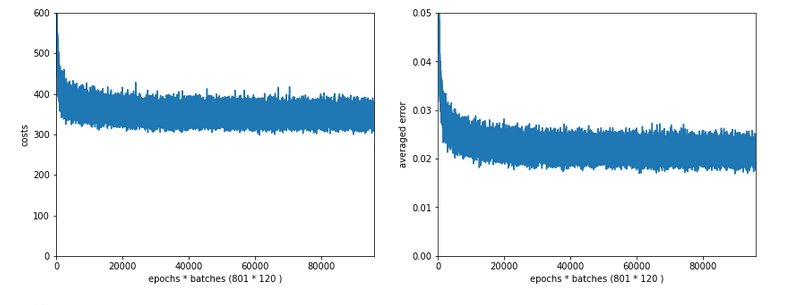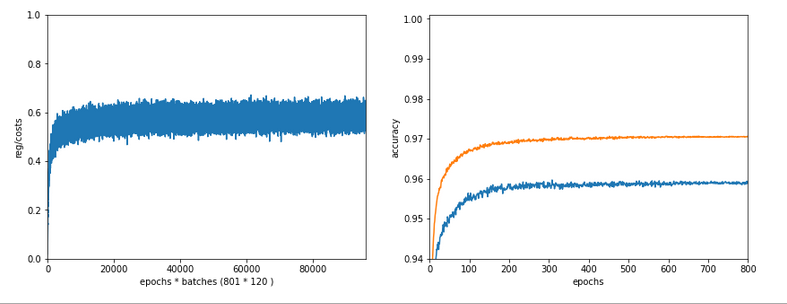OK, but in both cases we see a significant and systematic trend towards reduced accuracy values on the test data set with growing Lambda2-values > 0.2 for our chosen mini-batch size (500 samples).

# Conclusion

We learned a bit about the impact of regularization today. Whatever the exact Lambda2-value - in the end the contribution of a regularization term becomes a significant part of the total loss of a mini-batch when we approached the total cost minimum. However, the factor Lambda2 must be chosen with a reasonable size to get an impact of regularization on the final minimum position in the weight-space! But then it will help to improve accuracy on general input data in comparison to overfitted solutions!

But we also saw that there is some balance to take care of: For an optimum of generalization AND accuracy you should neither make Lambda2 too small nor too big. In our case Lambda2 = 0.2 seems to be a reasonable and good choice. Might be different with other datasets.

All in all studying the impact of a variation of achieved accuracy with the factor for a Ridge regularization term seems to be a good investment of time in ML projects. We shall come back to this point already in the next articles of this series.

In the next article

A simple Python program for an ANN to cover the MNIST dataset – XIV – cluster detection in feature space

we shall start to work on cluster detection in the feature space of the MNIST data before using gradient descent.

# A simple Python program for an ANN to cover the MNIST dataset – XI – confusion matrix

Welcome back to my readers who followed me through the (painful?) process of writing a Python class to simulate a "Multilayer Perceptron" [MLP]. The pain in my case resulted from the fact that I am still a beginner in Machine Learning [ML] and Python. Nevertheless, I hope that we have meanwhile acquired some basic understanding of how a MLP works and "learns". During the course of the last articles we had a close look at such nice things as "forward propagation", "gradient descent", "mini-batches" and "error backward propagation". For the latter I gave you a mathematical description to grasp the background of the matrix operations involved.

Where do we stand after 10 articles and a PDF on the math?

We have a working code

• with some parameters to control layers and node numbers, learning and momentum rates and regularization,
• with many dummy parts for other output and activation functions than the sigmoid function we used so far,
• with prepared code fragments for applying MSE instead of "Log Loss" as a cost function,
• and with dummy parts for handling different input datasets than the MNIST example.

The code is not yet optimized; it includes e.g. many statements for tests which we should eliminate or comment out. A completely open conceptual aspect is the optimization of the adaption of the learning rate; it is very primitive so far. We also need an export/import functionality to be able to perform training with a series of limited epoch numbers per run. We also should save the weights and accuracy data after a fixed epoch interval to be able to analyze a bit more after training. Another idea - though probably costly - is to even perform intermediate runs on the test data set an get some information on the development of the averaged error on the test data set.

Despite all these deficits, which we need to cover in some more articles, we are already able to perform an insightful task - namely to find out with which numbers and corresponding images of the MNIST data set our MLP has problems with. This leads us to the topics of a confusion matrix and other measures for the accuracy of our algorithm.

However, before we look at these topics, we first create some useful code, which we can save inside cells of the Jupyter notebook we maintain for testing our class "MyANN".

# Some functions to evaluate the prediction capability of our ANN after training

For further analysis we shall apply the following functions later on:

```# ------ predict results for all test data
# *************************
def predict_all_test_data():
size_set = ANN._X_test.shape

li_Z_in_layer_test  = [None] * ANN._n_total_layers
li_Z_in_layer_test = ANN._X_test

# Transpose input data matrix
ay_Z_in_0T       = li_Z_in_layer_test.T
li_Z_in_layer_test = ay_Z_in_0T
li_A_out_layer_test  = [None] * ANN._n_total_layers

# prediction by forward propagation of the whole test set
ANN._fw_propagation(li_Z_in = li_Z_in_layer_test, li_A_out = li_A_out_layer_test, b_print = False)
ay_predictions_test = np.argmax(li_A_out_layer_test[ANN._n_total_layers-1], axis=0)

# accuracy
ay_errors_test = ANN._y_test - ay_predictions_test
acc = (np.sum(ay_errors_test == 0)) / size_set
print ("total acc for test data = ", acc)

def predict_all_train_data():
size_set = ANN._X_train.shape

li_Z_in_layer_test  = [None] * ANN._n_total_layers
li_Z_in_layer_test = ANN._X_train
# Transpose
ay_Z_in_0T       = li_Z_in_layer_test.T
li_Z_in_layer_test = ay_Z_in_0T
li_A_out_layer_test  = [None] * ANN._n_total_layers

ANN._fw_propagation(li_Z_in = li_Z_in_layer_test, li_A_out = li_A_out_layer_test, b_print = False)
Result = np.argmax(li_A_out_layer_test[ANN._n_total_layers-1], axis=0)
Error = ANN._y_train - Result
acc = (np.sum(Error == 0)) / size_set
print ("total acc for train data = ", acc)

# Plot confusion matrix
# orginally from Runqi Yang;
# see https://gist.github.com/hitvoice/36cf44689065ca9b927431546381a3f7
def cm_analysis(y_true, y_pred, filename, labels, ymap=None, figsize=(10,10)):
"""
Generate matrix plot of confusion matrix with pretty annotations.
The plot image is saved to disk.
args:
y_true:    true label of the data, with shape (nsamples,)
y_pred:    prediction of the data, with shape (nsamples,)
filename:  filename of figure file to save
labels:    string array, name the order of class labels in the confusion matrix.
use `clf.classes_` if using scikit-learn models.
with shape (nclass,).
ymap:      dict: any -> string, length == nclass.
if not None, map the labels & ys to more understandable strings.
Caution: original y_true, y_pred and labels must align.
figsize:   the size of the figure plotted.
"""
if ymap is not None:
y_pred = [ymap[yi] for yi in y_pred]
y_true = [ymap[yi] for yi in y_true]
labels = [ymap[yi] for yi in labels]
cm = confusion_matrix(y_true, y_pred, labels=labels)
cm_sum = np.sum(cm, axis=1, keepdims=True)
cm_perc = cm / cm_sum.astype(float) * 100
annot = np.empty_like(cm).astype(str)
nrows, ncols = cm.shape
for i in range(nrows):
for j in range(ncols):
c = cm[i, j]
p = cm_perc[i, j]
if i == j:
s = cm_sum[i]
annot[i, j] = '%.1f%%\n%d/%d' % (p, c, s)
elif c == 0:
annot[i, j] = ''
else:
annot[i, j] = '%.1f%%\n%d' % (p, c)
cm = pd.DataFrame(cm, index=labels, columns=labels)
cm.index.name = 'Actual'
cm.columns.name = 'Predicted'
fig, ax = plt.subplots(figsize=figsize)
ax=sns.heatmap(cm, annot=annot, fmt='')
#plt.savefig(filename)

#
# Plotting
# **********
def plot_ANN_results():
num_epochs  = ANN._n_epochs
num_batches = ANN._n_batches
num_tot = num_epochs * num_batches

cshape = ANN._ay_costs.shape
print("n_epochs = ", num_epochs, " n_batches = ", num_batches, "  cshape = ", cshape )
tshape = ANN._ay_theta.shape
print("n_epochs = ", num_epochs, " n_batches = ", num_batches, "  tshape = ", tshape )

#sizing
fig_size = plt.rcParams["figure.figsize"]
fig_size = 12
fig_size = 5

# Two figures
# -----------
fig1 = plt.figure(1)
fig2 = plt.figure(2)

# first figure with two plot-areas with axes
# --------------------------------------------

ax1_1.plot(range(len(ANN._ay_costs)), ANN._ay_costs)
ax1_1.set_xlim (0, num_tot+5)
ax1_1.set_ylim (0, 1500)
ax1_1.set_xlabel("epochs * batches (" + str(num_epochs) + " * " + str(num_batches) + " )")
ax1_1.set_ylabel("costs")

ax1_2.plot(range(len(ANN._ay_theta)), ANN._ay_theta)
ax1_2.set_xlim (0, num_tot+5)
ax1_2.set_ylim (0, 0.15)
ax1_2.set_xlabel("epochs * batches (" + str(num_epochs) + " * " + str(num_batches) + " )")
ax1_2.set_ylabel("averaged error")

```

The first function "predict_all_test_data()" allows us to create an array with the predicted values for all test data. This is based on a forward propagation of the full set of test data; so we handle some relatively big matrices here. The second function delivers prediction values for all training data; the operations of propagation algorithm involve even bigger matrices here. You will nevertheless experience that the calculations are performed very quickly. Prediction is much faster than training!

The third function "cm_analysis()" is not from me, but taken from Github Gist; see below. The fourth function "plot_ANN_results()" creates plots of the evolution of the cost function and the averaged error after training. We come back to these functions below.

To be able to use these functions we need to perform some more imports first. The full list of statements which we should place in the first Jupyter cell of our test notebook now reads:

```import numpy as np
import numpy.random as npr
import math
import sys
import pandas as pd
from sklearn.datasets import fetch_openml
from sklearn.metrics import confusion_matrix
from scipy.special import expit
import seaborn as sns
from matplotlib import pyplot as plt
from matplotlib.colors import ListedColormap
import matplotlib.patches as mpat
import time
import imp
from mycode import myann
```

Note the new lines for the import of the "pandas" and "seaborn" libraries. Please inform yourself about the purpose of each library on the Internet.

# Limited Accuracy

In the last article we performed some tests which showed a thorough robustness of our MLP regarding the MNIST datatset. There was some slight overfitting, but playing around with hyper-parameters showed no extraordinary jump in "accuracy", which we defined to be the percentage of correctly predicted records in the test dataset.

In general we can say that an accuracy level of 95% is what we could achieve within the range of parameters we played around with. Regression regularization (Lambda2 > 0) had some positive impact. A structural change to a MLP with just one layer did NOT give us a real breakthrough regarding CPU-time consumption, but when going down to 50 or 30 nodes in the intermediate layer we saw at least some reduction by up to 25%. But then our accuracy started to become worse.

Whilst we did our tests we measured the ANN's "accuracy" by comparing the number of records for which our ANN did a correct prediction with the total number of records in the test data set. This is a global measure of accuracy; it averages over all 10 digits, i.e. all 10 classification categories. However, if we want to look a bit deeper into the prediction errors our MLP obviously produces it is, however, useful to introduce some more quantities and other measures of accuracy, which can be applied on the level of each output category.

# Measures of accuracy, related quantities and classification errors for a specific category

The following quantities and basic concepts are often used in the context of ML algorithms for classification tasks. Predictions of our ANN will not be error free and thus we get an accuracy less than 100%. There are different reasons for this - and they couple different output categories. In the case of MNIST the output categories correspond to the digits 0 to 9. Let us take a specific output category, namely the digit "5". Then there are two basic types of errors:

• The network may have predicted a "3" for a MNIST image record, which actually represents a "5" (according to the "y_train"-value for this record). This error case is called a "False Negative".
• The network may have predicted a "5" for a MNIST image record, which actually represents a "3" according to its "y_train"-value. This error case is called a "False Positive".

Both cases mark some difference between an actual and predicted number value for a MNIST test record. Technically, "actual" refers to the number value given by the related record in our array "ANN._y_test". "Predicted" refers to the related record in an array "ay_prediction_test", which our function "predict_all_test_data()" returns (see the code above).

Regarding our example digit "5" we obviously can distinguish between the following quantities:

• AN : The total number of all records in the test data set which actually correspond to our digit "5".
• TP: The number of "True Positives", i.e. the number of those cases correctly detected as "5"s.
• FP: The number of "False Positives", i.e. the number of those cases where our ANN falsely predicts a "5".
• FN: The number of "False Negatives", i.e. the number of those cases where our ANN falsely predicts another digit than "5", but where it actually should predict a "5".

Then we can calculate the following ratios which all somehow measure "accuracy" for a specific output category:

• Precision:
TP / (TP + FP)
• Recall:
TP / ( TP + FN))
• Accuracy:
TP / AN
• F1:
TP / ( TP + 0.5*(FN + TP) )

A careful reader will (rightly) guess that the quantity "recall" corresponds to what we would naively define as "accuracy" - namely the ratio TP/AN.
From its definition it is clear that the quantity "F1" gives us a weighted average between the measures "precision" and "recall".

How can we get these numbers for all 10 categories from our MLP after training ?

# Confusion matrix

When we want to analyze our basic error types per category we need to look at the discrepancy between predicted and actual data. This suggests a presentation in form of a matrix with all for all possible category values both in x- and y-direction. The cells of such a matrix - e.g. a cell for an actual "5" and a predicted "3" - could e.g. be filled with the corresponding FN-number.

We will later on develop our own code to solve the task of creating and displaying such a matrix. But there is a nice guy called Runqi Yang who shared some code for precisely this purpose on GitHub Gist; see https://gist.github.com/hitvoice/36c...
We can use his suggested code as it is in our context. We have already presented it above in form of the function "cm_analysis()", which uses the pandas and seaborn libraries.

After a training run with the following parameters

```try:
ANN = myann.MyANN(my_data_set="mnist_keras", n_hidden_layers = 2,
ay_nodes_layers = [0, 70, 30, 0],
n_nodes_layer_out = 10,
my_loss_function = "LogLoss",
n_size_mini_batch = 500,
n_epochs = 1800,
n_max_batches = 2000,  # small values only for test runs
lambda2_reg = 0.2,
lambda1_reg = 0.0,
vect_mode = 'cols',
learn_rate = 0.0001,
decrease_const = 0.000001,
mom_rate   = 0.00005,
shuffle_batches = True,
print_period = 50,
figs_x1=12.0, figs_x2=8.0,
legend_loc='upper right',
b_print_test_data = True
)
except SystemExit:
print("stopped")
```

When I studied the last plot for a while I found it really instructive. Each of its cell outside the diagonal obviously contains the number of "False Negative" records for these two specific category values - but with respect to actual value.

What more do we learn from the matrix? Well, the numbers in the cells on the diagonal, in a row and in a column are related to our quantities TP, FN and FP:

• Cells on the diagonal: For the diagonal we should find many correct "True Positive" values compared to the actual correct MNIST digits. (At least if all numbers are reasonably distributed across the MNIST dataset). We see that this indeed is the case. The ration of "True Positives" and the "Actual Positives" is given as a percentage and with the related numbers inside the respective cells on the diagonal.
• Cells of a row: The values in the cells of a row (without the cell on the diagonal) of the displayed matrix give us the numbers/ratios for "False Negatives" - with respect to the actual value. If you sum up the individual FN-numbers you get the total number of "False negatives", which of course is the difference between the total number AN and the number TP for the actual category.
• Cells of a column: The column cells contain the numbers/ratios for "False Positives" - with respect to the predicted value. If you sum up the individual FN-numbers you get the total number of "False Positives" with respect to the predicted column value.

So, be a bit careful: A FN value with respect to an actual row value is a FP value with respect to the predicted column value - if the cell is one outside the diagonal!

All ratios are calculated with respect to the total actual numbers of data records for a specific category, i.e. a digit.

Looking closely we detect that our code obviously has some problems with distinguishing pictures of "5"s with pictures of "3"s, "6"s and "8"s. The same is true for "8"s and "3"s or "2s". Also the distinction between "9"s, "3"s and "4"s seems to be difficult sometimes.

# Does the confusion matrix change due to random initial weight values and mini-batch-shuffling?

We have seen already that statistical variations have no big impact on the eventual accuracy when training converges to points in the parameter-space close to the point for the minimum of the overall cost-function. Statistical effects between to training runs stem in our case from statistically chosen initial values of the weights and the changes to our mini-batch composition between epochs. But as long as our training converges (and ends up in a global minimum) we should not see any big impact on the confusion matrix. And indeed a second run leads to: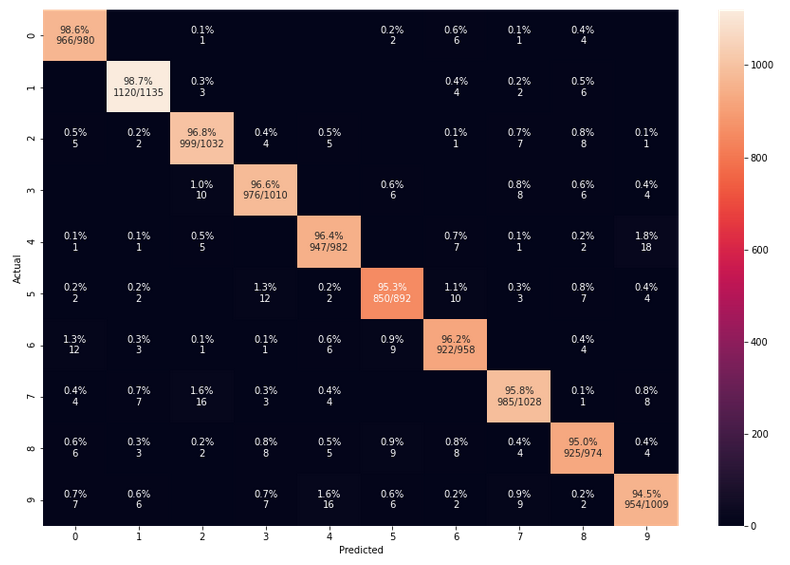The values are pretty close to those of the first run.

# Precision, Recall values per digit category and our own confusion matrix

Ok, we now can look at the nice confusion matrix plot and sum up all the values in a row of the confusion matrix to get the total FN-number for the related actual digit value. Or sum up the entries in a column to get the total FP-number. But we want to calculate these values from the ANN's prediction results without looking at a plot and summation handwork. In addition we want to get the data of the confusion matrix in our own Numpy matrix array independently of foreign code. The following box displays the code for two functions, which are well suited for this task:

```# A class to print in color and bold
class color:
PURPLE = '\033[95m'
CYAN = '\033[96m'
DARKCYAN = '\033[36m'
BLUE = '\033[94m'
GREEN = '\033[92m'
YELLOW = '\033[93m'
RED = '\033[91m'
BOLD = '\033[1m'
UNDERLINE = '\033[4m'
END = '\033[0m'

def acc_values(ay_pred_test, ay_y_test):
ay_x = ay_pred_test
ay_y = ay_y_test
# -----
#- dictionary for all false positives for all 10 digits
fp = {}
fpnum = {}
irg = range(10)
for i in irg:
key = str(i)
xfpi = np.where(ay_x==i)
fpi = np.zeros((10000, 3), np.int64)

n = 0
for j in xfpi:
if ay_y[j] != i:
row = np.array([j, ay_x[j], ay_y[j]])
fpi[n] = row
n+=1

fpi_real   = fpi[0:n]
fp[key]    = fpi_real
fpnum[key] = fp[key].shape

#- dictionary for all false negatives for all 10 digits
fn = {}
fnnum = {}
irg = range(10)
for i in irg:
key = str(i)
yfni = np.where(ay_y==i)
fni = np.zeros((10000, 3), np.int64)

n = 0
for j in yfni:
if ay_x[j] != i:
row = np.array([j, ay_x[j], ay_y[j]])
fni[n] = row
n+=1

fni_real = fni[0:n]
fn[key] = fni_real
fnnum[key] = fn[key].shape

#- dictionary for all true positives for all 10 digits
tp = {}
tpnum = {}
actnum = {}
irg = range(10)
for i in irg:
key = str(i)
ytpi = np.where(ay_y==i)
actnum[key] = ytpi.shape
tpi = np.zeros((10000, 3), np.int64)

n = 0
for j in ytpi:
if ay_x[j] == i:
row = np.array([j, ay_x[j], ay_y[j]])
tpi[n] = row
n+=1

tpi_real = tpi[0:n]
tp[key] = tpi_real
tpnum[key] = tp[key].shape

#- We create an array for the precision values of all 10 digits
ay_prec_rec_f1 = np.zeros((10, 9), np.int64)
print(color.BOLD + "Precision, Recall, F1, Accuracy, TP, FP, FN, AN" + color.END +"\n")
print(color.BOLD + "i  ", "prec  ", "recall  ", "acc    ", "F1       ", "TP    ",
"FP    ", "FN    ", "AN" + color.END)
for i in irg:
key = str(i)
tpn = tpnum[key]
fpn = fpnum[key]
fnn = fnnum[key]
an  = actnum[key]
precision = tpn / (tpn + fpn)
prec = format(precision, '7.3f')
recall = tpn / (tpn + fnn)
rec = format(recall, '7.3f')
accuracy = tpn / an
acc = format(accuracy, '7.3f')
f1 = tpn / ( tpn + 0.5 * (fnn+fpn) )
F1 = format(f1, '7.3f')
TP = format(tpn, '6.0f')
FP = format(fpn, '6.0f')
FN = format(fnn, '6.0f')
AN = format(an,  '6.0f')

row = np.array([i, precision, recall, accuracy, f1, tpn, fpn, fnn, an])
ay_prec_rec_f1[i] = row
print (i, prec, rec, acc, F1, TP, FP, FN, AN)

return tp, tpnum, fp, fpnum, fn, fnnum, ay_prec_rec_f1

def create_cf(ay_fn, ay_tpnum):
''' fn: array with false negatives row = np.array([j, x[j], y[j]])
'''
cf = np.zeros((10, 10), np.int64)
rgi = range(10)
rgj = range(10)
for i in rgi:
key = str(i)
fn_i = ay_fn[key][ay_fn[key][:,2] == i]
for j in rgj:
if j!= i:
fn_ij = fn_i[fn_i[:,1] == j]
#print(i, j, fn_ij)
num_fn_ij = fn_ij.shape
cf[i,j] = num_fn_ij
if j==i:
cf[i,j] = ay_tpnum[key]

cols=["0", "1", "2", "3", "4", "5", "6", "7", "8", "9"]
df = pd.DataFrame(cf, columns=cols, index=cols)
# print( "\n", df, "\n")
# df.style

return cf, df

```

The first function takes a array with prediction values (later on provided externally by our "ay_predictions_test") and compares its values with those of an y_test array which contains the actual values (later provided externally by our "ANN._y_test"). Then it uses array-slicing to create new arrays with information on all error records, related indices and the confused category values. Eventually, the function determines the numbers for AN, TP, FP and FN (per digit category) and prints the gathered information. It also returns arrays with information on records which are "True Positives", "False Positives", "False Negatives" and the various numbers.

The second function uses array-slicing of the array which contains all information on the "False Negatives" to reproduce the confusion matrix. It involves Pandas to produce a styled output for the matrix.

Now you can run the above code and the following one in Jupyter cells - of course, only after you have completed a training and a prediction run: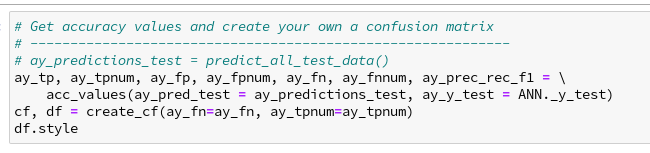For my last run I got the following data: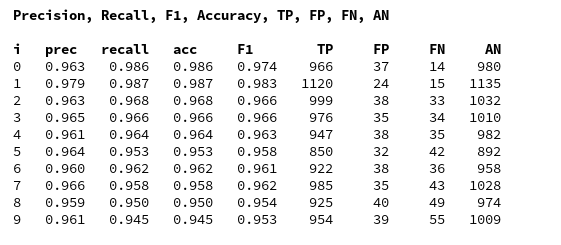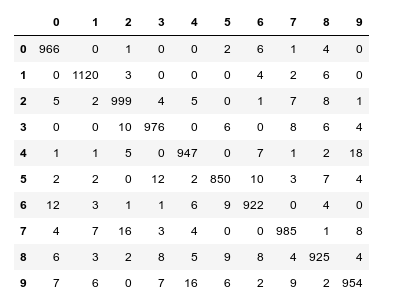We again see that especially "5"s and "9"s have a problem with FNs. When you compare the values of the last printed matrix with those in the plot of the confusion matrix above, you will see that our code produces the right FN/FP/TP-values. We have succeeded in producing our own confusion matrix - and we have all values directly available in our own Numpy arrays.

# Some images of "4"-digits with errors

We can use the arrays which we created with functions above to get a look at the images. We use the function "plot_digits()" of Aurelien Geron at handson-ml2 chapter 03 on classification to plot several images in a series of rows and columns. The code is pretty easy to understand; at its center we find the matplotlib-function "imshow()", which we have already used in other ML articles.

We again perform some array-slicing of the arrays our function "acc_values()" (see above) produces to identify the indices of images in the "X_test"-dataset we want to look at. We collect the first 50 examples of "true positive" images of the "4"-digit, then we take the "false positives" of the 4-digit and eventually the "fales negative" cases. We then plot the images in this order:

```def plot_digits(instances, images_per_row=10, **options):
size = 28
images_per_row = min(len(instances), images_per_row)
images = [instance.reshape(size,size) for instance in instances]
n_rows = (len(instances) - 1) // images_per_row + 1
row_images = []
n_empty = n_rows * images_per_row - len(instances)
images.append(np.zeros((size, size * n_empty)))
for row in range(n_rows):
rimages = images[row * images_per_row : (row + 1) * images_per_row]
row_images.append(np.concatenate(rimages, axis=1))
image = np.concatenate(row_images, axis=0)
plt.imshow(image, cmap = mpl.cm.binary, **options)
plt.axis("off")

ay_tp, ay_tpnum, ay_fp, ay_fpnum, ay_fn, ay_fnnum, ay_prec_rec_f1 = \
acc_values(ay_pred_test = ay_predictions_test, ay_y_test = ANN._y_test)

idx_act = str(4)

# fetching the true positives
num_tp = ay_tpnum[idx_act]
idx_tp = ay_tp[idx_act][:,]
idx_tp = idx_tp[:,0]
X_test_tp = ANN._X_test[idx_tp]

# fetching the false positives
num_fp = ay_fpnum[idx_act]
idx_fp = ay_fp[idx_act][:,]
idx_fp = idx_fp[:,0]
X_test_fp = ANN._X_test[idx_fp]

# fetching the false negatives
num_fn = ay_fnnum[idx_act]
idx_fn = ay_fn[idx_act][:,]
idx_fn = idx_fn[:,0]
X_test_fn = ANN._X_test[idx_fn]

# plotting
# +++++++++++
plt.figure(figsize=(12,12))

# plotting the true positives
# --------------------------
plt.subplot(321)
plot_digits(X_test_tp[0:25], images_per_row=5 )
plt.subplot(322)
plot_digits(X_test_tp[25:50], images_per_row=5 )

# plotting the false positives
# --------------------------
plt.subplot(323)
plot_digits(X_test_fp[0:25], images_per_row=5 )
plt.subplot(324)
plot_digits(X_test_fp[25:], images_per_row=5 )

# plotting the false negatives
# ------------------------------
plt.subplot(325)
plot_digits(X_test_fn[0:25], images_per_row=5 )
plt.subplot(326)
plot_digits(X_test_fn[25:], images_per_row=5 )

```

The first row of the plot shows the (first) 50 "True Positives" for the "4"-digit images in the MNIST test data set. The second row shows the "False Positives", the third row the "False Negatives".

Very often you can guess why our MLP makes a mistake. However, in some cases we just have to acknowledge that the human brain is a much better pattern recognition machine than a stupid MLP 🙂 .

# Conclusion

With the help of a "confusion matrix" it is easy to find out for which MNIST digit-images our algorithm has major problems. A confusion matrix gives us the necessary numbers of those digits (and their images) for which the MLP wrongly predicts "False Positives" or "False Negatives".

We have also seen that there are three quantities - precision, recall, F1 - which are useful to describe the accuracy of a classification algorithm per classification category.

We have written some code to collect all necessary information about "confused" images into our own Numpy arrays after training. Slicing of Numpy arrays proved to be useful, and matplotlib helped us to visualize examples of the wrongly classified MNIST digit-images.

In the next article
A simple program for an ANN to cover the Mnist dataset – XII – accuracy evolution, learning rate, normalization
we shall extract some more information on the evolution of accuracy during training. We shall also make use of a "clustering" technique to reduce the number of input nodes.